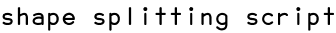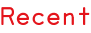RSS
shape splitting script
From:  Oleg
Date:  21. December 2011, 08:48

I was wondering if it's easy to create a script which would split large rectangle into user defined number of rectangles of various dimensions (height and width), but the same square area? The trick is that the new rectangles should recreate the area and dimensions of the big rectangle when stacked.

Would be nice if the script had following features:

1. The output is the original big rectangle sliced into smaller rectangles of equal square area.
2. User defines number of rectangles.
3. User defines the variations in square areas of the rectangles (% of calculated equal square areas: -% 100% +%).
4. User can control minimum and maximum width and height of generated rectangles.
5. A "randomize" button which would generate various combinations of stacked rectangles.
6. And finally would be nice to have a checkbox which would allow to prevent having duplicate rectangle.

Not sure if this is achievable or not, but it would be cool to have such script. This script would allow quickly generate a random and uneven grid for some content (placed into rectangles) for a given space (the big rectangle).

Scripts
08.08.14, 15:24
15.05.14, 14:23
02.03.14, 19:16
18.11.13, 14:48
22.03.13, 03:05
22.02.13, 15:45
Posts
10.01.17, 16:37
19.02.16, 06:03
19.02.16, 06:00
17.01.16, 11:00
12.01.16, 13:10
25.11.15, 08:19
Script of the Moment